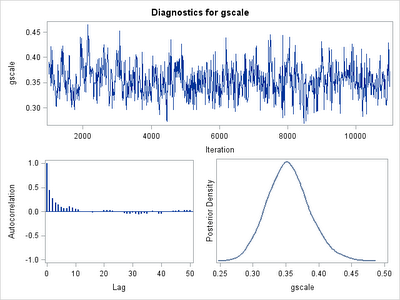## Tuesday, October 4, 2011

### Example 9.8: New stuff in SAS 9.3-- Bayesian random effects models in Proc MCMCRounding off our reports on major new developments in SAS 9.3, today we'll talk about proc mcmc and the random statement.

Stand-alone packages for fitting very general Bayesian models using Markov chain Monte Carlo (MCMC) methods have been available for quite some time now. The best known of these are BUGS and its derivatives WinBUGS (last updated in 2007) and OpenBUGS . There are also some packages available that call these tools from R.

Today we'll consider a relatively simple model: Clustered Poisson data where cluster means are a constant plus a cluster-specific exponentially-distributed random effect. To be clear:
y_ij ~ Poisson(mu_i)
log(mu_i) = B_0 + r_i
r_i ~ Exponential(lambda)
Of course in Bayesian thinking all effects are random-- here we use the term in the sense of cluster-specific effects.

SAS
Several SAS procedures have a bayes statement that allow some specific models to be fit. For example, in Section 6.6 and example 8.17, we show Bayesian Poisson and logistic regression, respectively, using proc genmod. But our example today is a little unusual, and we could not find a canned procedure for it. For these more general problems, SAS has proc mcmc, which in SAS 9.3 allows random effects to be easily modeled.

We begin by generating the data, and fitting the naive (unclustered) model. We set B_0 = 1 and lambda = 0.4. There are 200 clusters of 10 observations each, which we might imagine represent 10 students from each of 200 classrooms.
`data test2;truebeta0 = 1;randscale = .4;call streaminit(1944);  do i = 1 to 200;    randint = rand("EXPONENTIAL") * randscale;    do ni = 1 to 10;      mu = exp(truebeta0  + randint);       y = rand("POISSON", mu);      output;    end;  end;run;proc genmod data = test2;model y = / dist=poisson;run;                      Standard       Wald 95%     Parameter  Estimate     Error   Confidence LimitsIntercept    1.4983    0.0106    1.4776    1.5190`

Note the inelegant SAS syntax for fitting an intercept-only model. The result is pretty awful-- 50% bias with respect to the global mean. Perhaps we'll do better by acknowledging the clustering. We might try that with normally distributed random effects in proc glimmix.
`proc glimmix data = test2 method=laplace;class i;model y = / dist = poisson solution;random int / subject = i type = un;run;   Cov                               Standard   Parm       Subject    Estimate       Error   UN(1,1)    i            0.1682     0.01841                       StandardEffect     Estimate     Error  t Value  Pr > |t|Intercept    1.3805   0.03124    44.20    <.0001`

No joy-- still a 40% bias in the estimated mean. And the variance of the random effects is biased by more than 50%! Let's try fitting the model that generated the data.
`proc mcmc data=test2 nmc=10000 thin=10 seed=2011;parms fixedint 1 gscale 0.4;prior fixedint ~ normal(0, var = 10000);prior gscale ~ igamma(.01 , scale = .01 ) ;random rint ~ gamma(shape=1, scale=gscale) subject = i initial=0.0001;mu = exp(fixedint + rint);model y ~ poisson(mu);run;`

The key points of the proc mcmc statement are nmc, the total number of Monte Carlo iterations to perform, and thin, which includes only every nth sample for inference. The prior and model statements are fairly obvious; we note that in more complex models, parameters that are listed within a single prior statement are sampled as a block. We're placing priors on the fixed (shared) intercept and the scale of the exponential. The mu line is actually just a programming statement-- it uses the same syntax as data step programming.
The newly available statement is random. The syntax here is similar to those for the other priors, with the addition of the subject option, which generates a unique parameter for each level of the subject variable. The random effects themselves can be used in later statements, as shown, to enter into data distributions. A final note here is that the exponential distribution isn't explicitly available, but since the gamma distribution with shape fixed at 1 defines the exponential, this is not a problem. Here are the key results.
`           Posterior Summaries                               Standard          Parameter        N     Mean Deviation    fixedint      1000   1.0346    0.0244    gscale        1000   0.3541    0.0314             Posterior Intervals Parameter    Alpha        HPD Interval fixedint     0.050      0.9834      1.0791 gscale       0.050      0.2937      0.4163`

The 95% HPD regions include the true values of the parameters and the posterior means are much less biased than in the model assuming normal random effects.

As usual, MCMC models should be evaluated carefully for convergence and coverage. In this example, I have some concerns (see default diagnostic figure above) and if it were real data I would want to do more.

R
The CRAN task view on Bayesian Inference includes a summary of tools for general and model-specific MCMC tools. However, there is nothing like proc mcmc in terms of being a general and easy to use tool that is native to R. The nearest options are to use R front ends to WinBUGS/OpenBUGS (R2WinBUGS) or JAGS (rjags). (A brief worked example of using rjags was posted last year by John Myles White.) Alternatively, with some math and a little sweat, the mcmc package would also work. We'll explore an approach through one or more of these packages in a later entry, and would welcome a collaboration from anyone who would like to take that on.